# AI, morality, ethics and metaethics

Next Sunday I will be debating AI ethics at Battle of Ideas. Here is a podcast where I talk AI, morality and ethics: https://soundcloud.com/institute-of-ideas/battle-cry-anders-sandberg-on-ethical-ai

# What distinguishes morals from ethics?

There is actually a shocking confusion about what the distinction between morals and ethics is. Differen.com says ethics is about rules of conduct produced by an external source while morals are an individual’s own principles of right and wrong. Grammarist.com says morals are principles on which one’s own judgement of right and wrong are based (abstract, subjective and personal), ethics are the principles of right conduct (practical, social and objective). Ian Welsh gives a soundbite: “morals are how you treat people you know.  Ethics are how you treat people you don’t know.” Paul Walker and Terry Lovat say ethics leans towards decisions based on individual character and subjective understanding of right and wrong, while morals is about widely shared communal or societal norms – here ethics is individual assessment of something being good or bad, while morality is inter-subjective community assessment.

Wikipedia distinguishes between ethics as a research field and the common human ability to think critically about moral values and direct actions appropriately, or a particular persons principles of values. Morality is the differentiation between things that are proper and improper, as well as a body of standards and principles in derived from a code of conduct in some philosophy, religion or culture… or derived from a standard a person believes to be universal.

Dictionary.com regards ethics as a system of moral principles, the rules of conduct recognized in some human environment, an individual’s moral principles (and the branch of philosophy). Morality is about conforming to the rules of right conduct, having moral quality or character, a doctrine or system of morals and a few other meanings. The Cambridge dictionary thinks ethics is the study of what is right or wrong, or the set of beliefs about it, while morality is a set of personal or social standards for good/bad behavior and character.

And so on.

I think most people try to include the distinction between shared systems of conduct and individual codes, and the distinction between things that are subjective, socially agreed on, and maybe objective. Plus that we all agree on that ethics is a philosophical research field.

# My take on it

I like to think of it as a AI issue. We have a policy function$\pi(s,a)$ that maps states and action pairs to a probability of acting that way; this is set using a value function$Q(s)$ where various states are assigned values. Morality in my sense is just the policy function and maybe the value function: they have been learned through interacting with the world in various ways.

Ethics in my sense is ways of selecting policies and values. We are able to not only change how we act but also how we evaluate things, and the information that does this change is not just reward signals that update value function directly, but also knowledge about the world, discoveries about ourselves, and interactions with others – in particular ideas that directly change the policy and value functions.

When I realize that lying rarely produces good outcomes (too much work) and hence reduce my lying, then I am doing ethics (similarly, I might be convinced about this by hearing others explain that lying is morally worse than I thought or convincing me about Kantian ethics). I might even learn that short-term pleasure is less valuable than other forms of pleasure, changing how I view sensory rewards.

Academic ethics is all about the kinds of reasons and patterns we should use to update our policies and values, trying to systematize them. It shades over into metaethics, which is trying to understand what ethics is really about (and what metaethics is about: it is its own meta-discipline, unlike metaphysics that has metametaphysics, which I think is its own meta-discipline).

I do not think I will resolve any confusion, but at least this is how I tend to use the terminology. Morals is how I act and evaluate, ethics is how I update how I act and evaluate, metaethics is how I try to think about my ethics.

# How much should we spread out across future scenarios?

Robin Hanson mentions that some people take him to task for working on one scenario (WBE) that might not be the most likely future scenario (“standard AI”); he responds by noting that there are perhaps 100 times more people working on standard AI than WBE scenarios, yet the probability of AI is likely not a hundred times higher than WBE. He also notes that there is a tendency for thinkers to clump onto a few popular scenarios or issues. However:

In addition, due to diminishing returns, intellectual attention to future scenarios should probably be spread out more evenly than are probabilities. The first efforts to study each scenario can pick the low hanging fruit to make faster progress. In contrast, after many have worked on a scenario for a while there is less value to be gained from the next marginal effort on that scenario.

This is very similar to my own thinking about research effort. Should we focus on things that are likely to pan out, or explore a lot of possibilities just in case one of the less obvious cases happens? Given that early progress is quick and easy, we can often get a noticeable fraction of whatever utility the topic has by just a quick dip. The effective altruist heuristic of looking at neglected fields also is based on this intuition.

# A model

But under what conditions does this actually work? Here is a simple model:

There are$N$ possible scenarios, one of which ($j$) will come about. They have probability$P_i$. We allocate a unit budget of effort to the scenarios:$\sum a_i = 1$. For the scenario that comes about, we get utility$\sqrt{a_j}$ (diminishing returns).

Here is what happens if we allocate proportional to a power of the scenarios,$a_i \propto P_i^\alpha$.$\alpha=0$ corresponds to even allocation, 1 proportional to the likelihood, >1 to favoring the most likely scenarios. In the following I will run Monte Carlo simulations where the probabilities are randomly generated each instantiation. The outer bluish envelope represents the 95% of the outcomes, the inner ranges from the lower to the upper quartile of the utility gained, and the red line is the expected utility.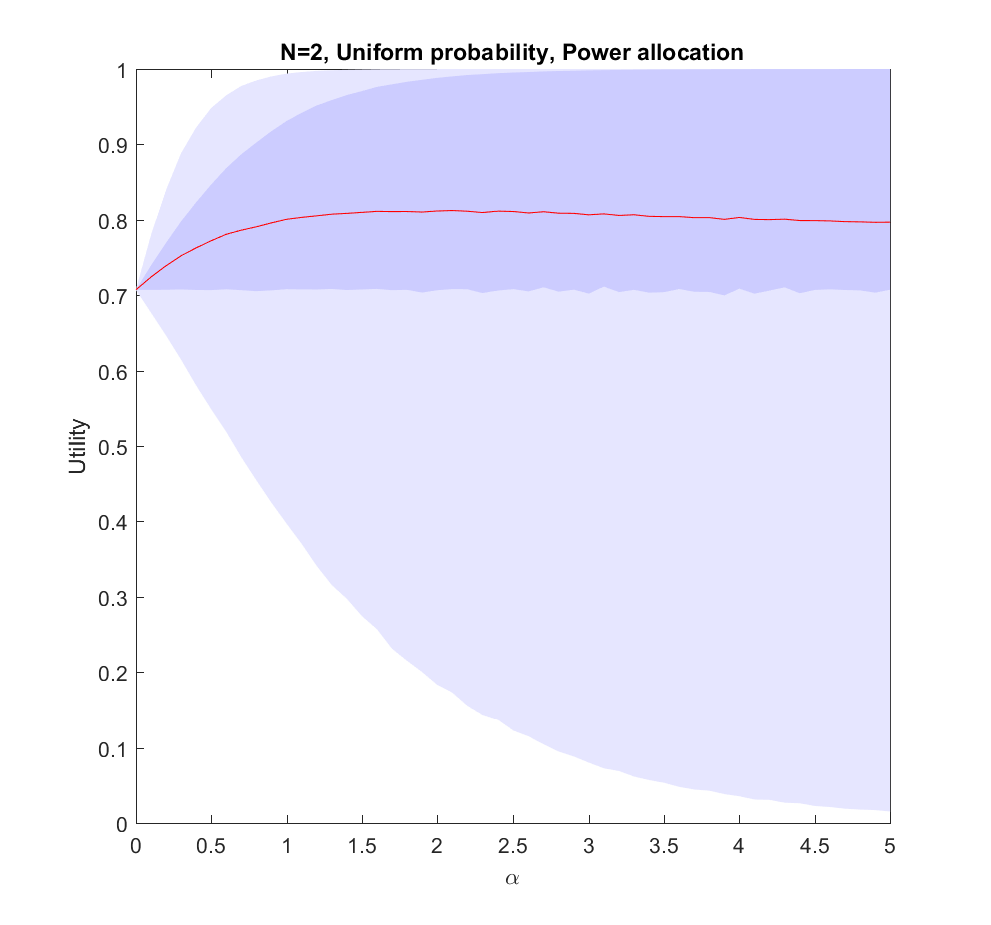Utility of allocating effort as a power of the probability of scenarios. Red line is expected utility, deeper blue envelope is lower and upper quartiles, lighter blue 95% interval.

This is the$N=2$ case: we have two possible scenarios with probability$p$ and$1-p$ (where$p$ is uniformly distributed in [0,1]). Just allocating evenly gives us$1/\sqrt{2}$ utility on average, but if we put in more effort on the more likely case we will get up to 0.8 utility. As we focus more and more on the likely case there is a corresponding increase in variance, since we may guess wrong and lose out. But 75% of the time we will do better than if we just allocated evenly. Still, allocating nearly everything to the most likely case means that one does lose out on a bit of hedging, so the expected utility declines slowly for large$\alpha$.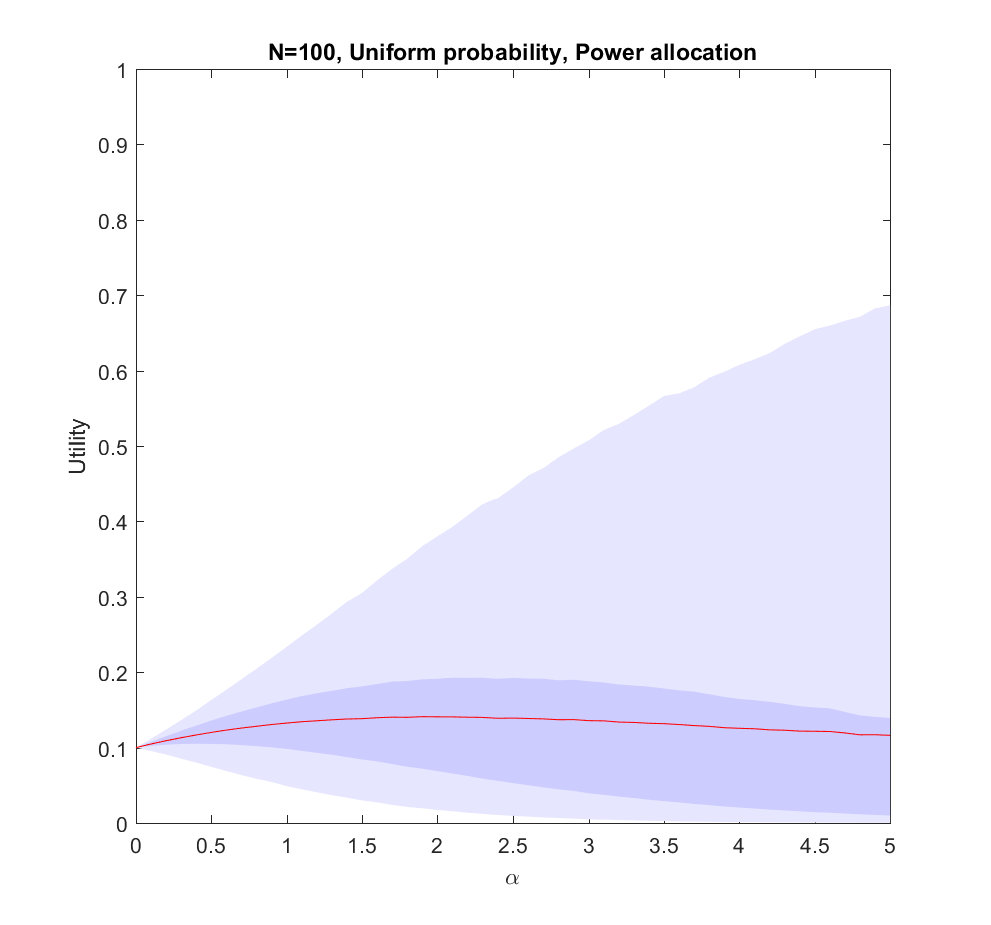Utility of allocating effort as a power of the probability of scenarios. Red line is expected utility, deeper blue envelope is lower and upper quartiles, lighter blue 95% interval. 100 possible scenarios, with uniform probability on the simplex.

The$N=100$ case (where the probabilities are allocated based on a flat Dirichlet distribution) behaves similarly, but the expected utility is smaller since it is less likely that we will hit the right scenario.

# What is going on?

This doesn’t seem to fit Robin’s or my intuitions at all! The best we can say about uniform allocation is that it doesn’t produce much regret: whatever happens, we will have made some allocation to the possibility. For large N this actually works out better than the directed allocation for a sizable fraction of realizations, but on average we get less utility than betting on the likely choices.

The problem with the model is of course that we actually know the probabilities before making the allocation. In reality, we do not know the likelihood of AI, WBE or alien invasions. We have some information, and we do have priors (like Robin’s view that$P_{AI} < 100 P_{WBE}$), but we are not able to allocate perfectly.  A more plausible model would give us probability estimates instead of the actual probabilities.

# We know nothing

Let us start by looking at the worst possible case: we do not know what the true probabilities are at all. We can draw estimates from the same distribution – it is just that they are uncorrelated with the true situation, so they are just noise.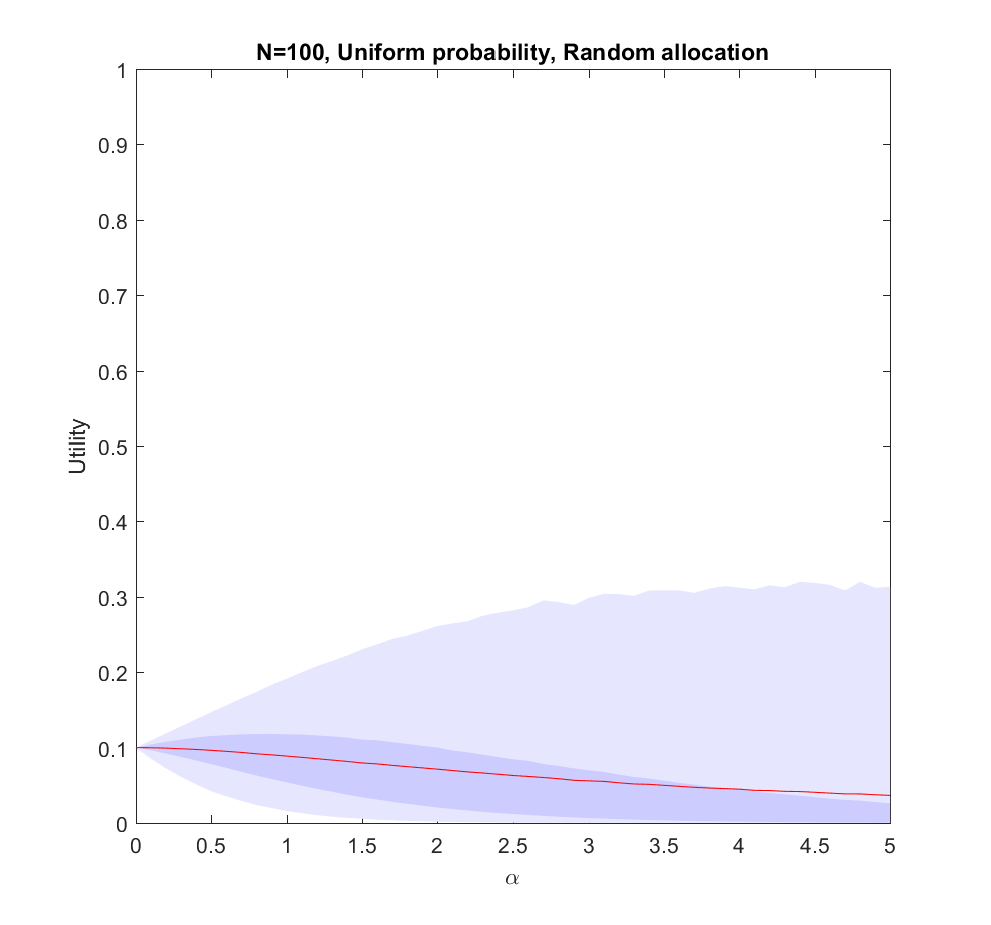Utility of allocating effort as a power of the probability of scenarios, but the probabilities are just random guesses. Red line is expected utility, deeper blue envelope is lower and upper quartiles, lighter blue 95% interval. 100 possible scenarios, with uniform probability on the simplex.

In this case uniform distribution of effort is optimal. Not only does it avoid regret, it has a higher expected utility than trying to focus on a few scenarios ($\alpha>0$). The larger N is, the less likely it is that we focus on the right scenario since we know nothing. The rationality of ignoring irrelevant information is pretty obvious.

Note that if we have to allocate a minimum effort to each investigated scenario we will be forced to effectively increase our$\alpha$ above 0. The above result gives the somewhat optimistic conclusion that the loss of utility compared to an even spread is rather mild: in the uniform case we have a pretty low amount of effort allocated to the winning scenario, so the low chance of being right in the nonuniform case is being balanced by having a slightly higher effort allocation on the selected scenarios. For high$\alpha$ there is a tail of rare big “wins” when we hit the right scenario that drags the expected utility upwards, even though in most realizations we bet on the wrong case. This is very much the hedgehog predictor story: ocasionally they have analysed the scenario that comes about in great detail and get intensely lauded, despite looking at the wrong things most of the time.

# We know a bit

We can imagine that knowing more should allow us to gradually interpolate between the different results: the more you know, the more you should focus on the likely scenarios.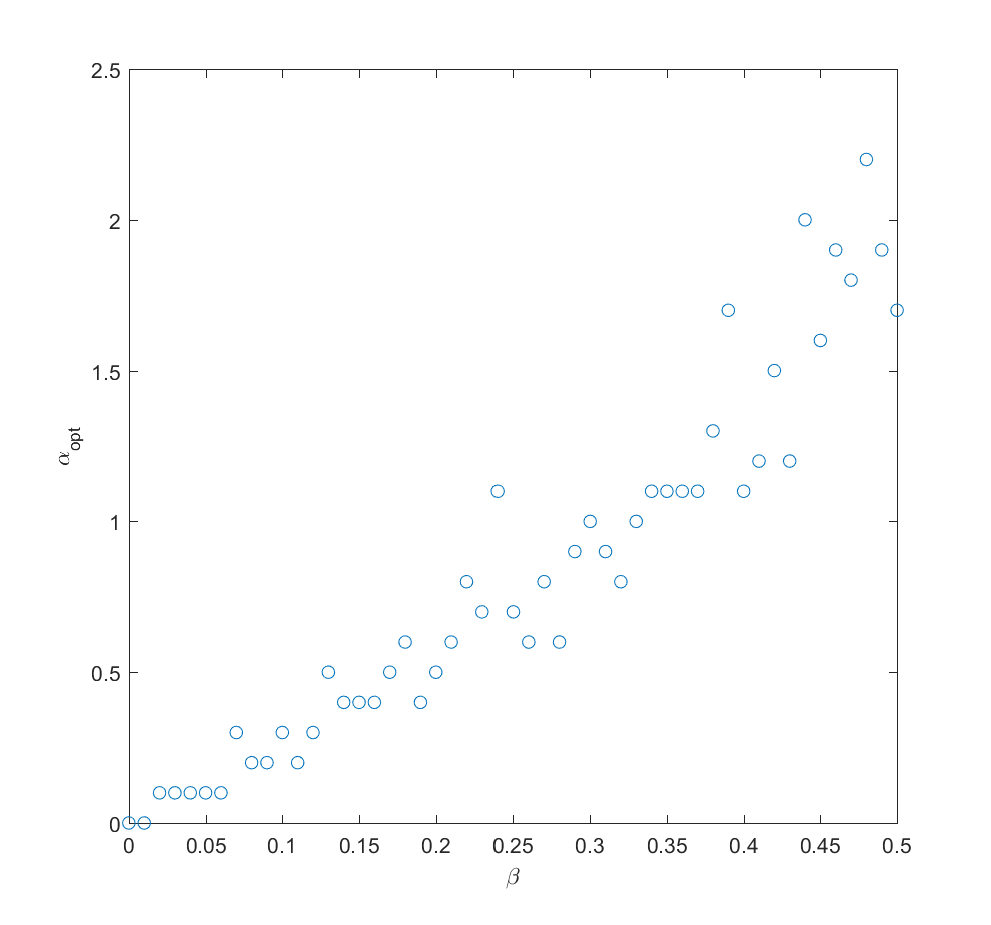Optimal alpha as a function of how much information we have about the true probabilities (noise due to Monte Carlo and discrete steps of alpha). N=2 (N=100 looks similar).

If we take the mean of the true probabilities with some randomly drawn probabilities (the “half random” case) the curve looks quite similar to the case where we actually know the probabilities: we get a maximum for$\alpha\approx 2$. In fact, we can mix in just a bit ($\beta$) of the true probability and get a fairly good guess where to allocate effort (i.e. we allocate effort as$a_i \propto (\beta P_i + (1-\beta)Q_i)^\alpha$ where$Q_i$ is uncorrelated noise probabilities). The optimal alpha grows roughly linearly with$\beta$,$\alpha_{opt} \approx 4\beta$ in this case.

# We learn

Adding a bit of realism, we can consider a learning process: after allocating some effort$\gamma$ to the different scenarios we get better information about the probabilities, and can now reallocate. A simple model may be that the standard deviation of noise behaves as$1/\sqrt{\tilde{a}_i}$ where$\tilde{a}_i$ is the effort placed in exploring the probability of scenario$i$. So if we begin by allocating uniformly we will have noise at reallocation of the order of$1/\sqrt{\gamma/N}$. We can set$\beta(\gamma)=\sqrt{\gamma/N}/C$, where$C$ is some constant denoting how tough it is to get information. Putting this together with the above result we get$\alpha_{opt}(\gamma)=\sqrt{2\gamma/NC^2}$. After this exploration, now we use the remaining$1-\gamma$ effort to work on the actual scenarios.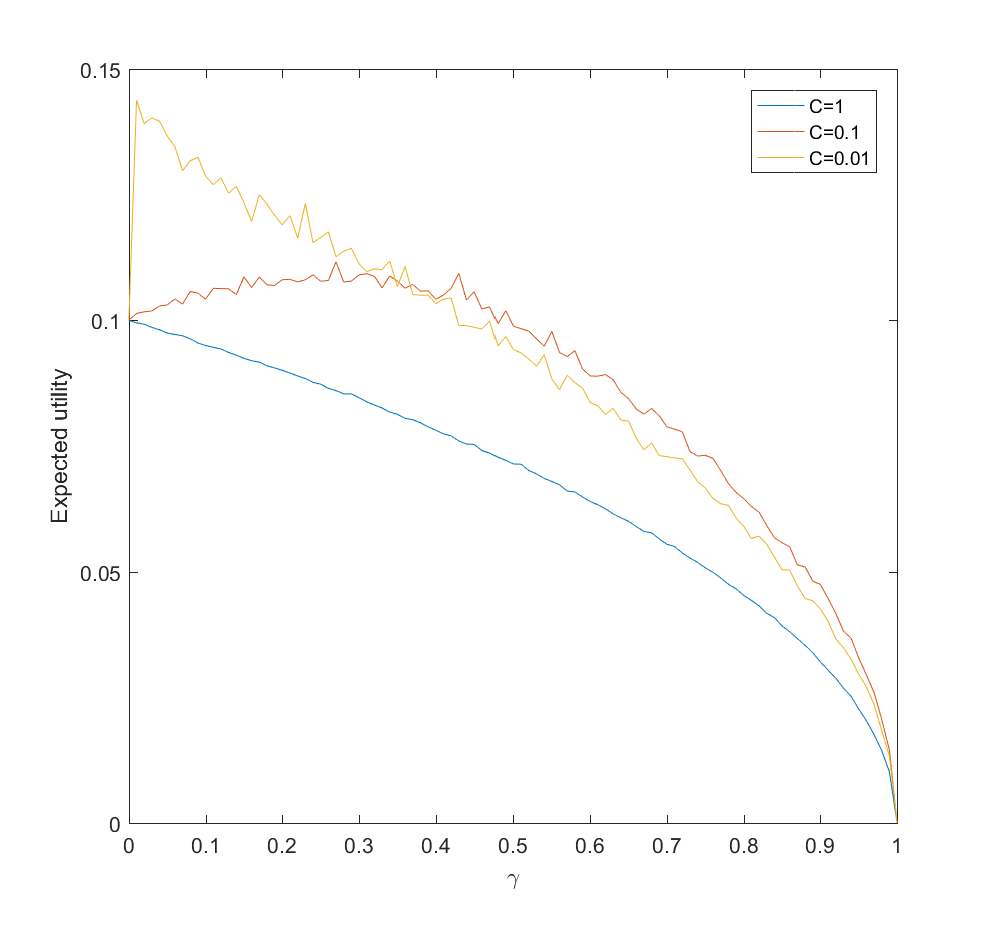Expected utility as a function of amount of probability-estimating effort (gamma) for C=1 (hard to update probabilities), C=0.1 and C=0.01 (easy to update). N=100.

This is surprisingly inefficient. The reason is that the expected utility declines as$\sqrt{1-\gamma}$ and the gain is just the utility difference between the uniform case$\alpha=0$ and optimal$\alpha_{opt}$, which we know is pretty small. If C is small (i.e. a small amount of effort is enough to figure out the scenario probabilities) there is an optimal nonzero$\gamma$. This optimum$\gamma$ decreases as C becomes smaller. If C is large, then the best approach is just to spread efforts evenly.

# Conclusions

So, how should we focus? These results suggest that the key issue is knowing how little we know compared to what can be known, and how much effort it would take to know significantly more.

If there is little more that can be discovered about what scenarios are likely, because our state of knowledge is pretty good, the world is very random,  or improving knowledge about what will happen will be costly, then we should roll with it and distribute effort either among likely scenarios (when we know them) or spread efforts widely (when we are in ignorance).

If we can acquire significant information about the probabilities of scenarios, then we should do it – but not overdo it. If it is very easy to get information we need to just expend some modest effort and then use the rest to flesh out our scenarios. If it is doable but costly, then we may spend a fair bit of our budget on it. But if it is hard, it is better to go directly on the object level scenario analysis as above. We should not expect the improvement to be enormous.

Here I have used a square root diminishing return model. That drives some of the flatness of the optima: had I used a logarithm function things would have been even flatter, while if the returns diminish more mildly the gains of optimal effort allocation would have been more noticeable. Clearly, understanding the diminishing returns, number of alternatives, and cost of learning probabilities better matters for setting your strategy.

In the case of future studies we know the number of scenarios are very large. We know that the returns to forecasting efforts are strongly diminishing for most kinds of forecasts. We know that extra efforts in reducing uncertainty about scenario probabilities in e.g. climate models also have strongly diminishing returns. Together this suggests that Robin is right, and it is rational to stop clustering too hard on favorite scenarios. Insofar we learn something useful from considering scenarios we should explore as many as feasible.

# The case for MarsOn practical Ethics I post about the goodness of being multi-planetary: is it rational to try to settle Mars as a hedge against existential risk?

The problem is not that it is absurd to care about existential risks or the far future (which was the Economist‘s unfortunate claim), nor that it is morally wrong to have a separate colony, but that there might be better risk reduction strategies with more bang for the buck.

One interesting aspect is that making space more accessible makes space refuges a better option. At some point in the future, even if space refuges are currently not the best choice, they may well become that. There are of course other reasons to do this too (science, business, even technological art).

So while existential risk mitigation right now might rationally aim at putting out the current brushfires and trying to set the long-term strategy right, doing the groundwork for eventual space colonisation seems to be rational.